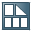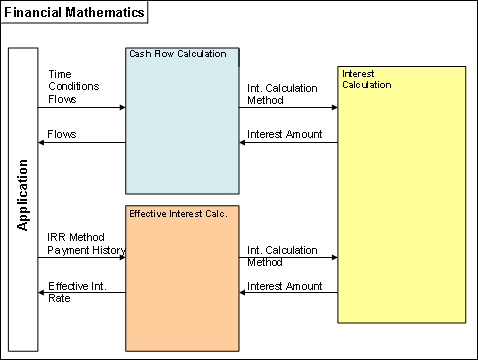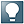Show TOC##Financial Mathematics (CA-FIM-FMA)### Purpose

This component provides financial mathematical functions that you need for the calculation of interest and effective interest. In addition, this component contains numerous general functions that are also useful in other situations.

At the center of the Financial Mathematics (FiMa) is the generation of payment flows that the system calculates on the basis of the conditions of a financial transaction and market data. These types of payment flows go into the calculation of interest and effective interest, but are also used by components for specific industries, for example, for overall bank controlling. There the payment flows are used, for example, to analyze the incoming and outgoing payments of a financial transaction over a long period of time that reaches into the future, and to estimate the related market risks.

Furthermore, financial mathematic methods are used in loans and securities management, money market trading , derivatives, leasing contracts, checking accounts, and other applications.

The Financial Mathematics component is available for all applications. This guarantees the standardized and consistent calculation of interest and effective interest.

The following chart shows the relationships between the main functions of financial mathematics:### Implementation Considerations

The component Financial Mathematics does not have its own Customizing for IMG activities.  However, you can store your own formulas for determining an interest rate in Table AT30. You can use these formulas when you edit conditions.

### Integration

The functions of the Financial Mathematics component are implemented as function modules and are available across all applications. Because the function modules belong to a single software level they can be used by other components without restrictions.The FIMAtransaction is available for testing some financial mathematical methods.   You can include the FIMA transaction in the area menu manually. It is not included in the area menu of the standard system (SAP Easy Access screen).

### Features

The Financial Mathematics component includes the following functions:

Interest Calculation

The system calculates interest amounts based on parameters. These include the relevant periods, interest rates and interest calculation methods. For example, checking accounts call up interest calculation for each period settlement.Cash Flow Calculation

The system generates payment flows, adjusts reference interest rates, and calculates interest and repayment.Effective Interest Calculation

The system calculates the effective interest of a transaction based on the payment histories that the calling application transfers from the payment flows. For example, effective interest calculation determines the cost of a loan and enables a comparison of the costs of different financial transactions.General Functions

Financial Mathematics provides general functions for different financial mathematical areas.  These include determination of the number of days in a period, the rounding of numerical values, and the execution of the numerical iterative procedure.